## 求两个大数的精确加减法，a=46328648326846835684657654876576657652364652442384, b=47,提示：不可以用java类，要用数组的方法来做，一位一位的加处理进位。

0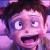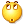都说了这么详细了
0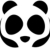```        int[] max={4,6,3,2,8,6,4,8,3,2,6,8,4,6,8,3,5,6,8,4,6,5,7,6,5,4,8,7,6,5,7,6,6,5,7,6,5,2,3,6,4,6,5,2,4,4,2,3,8,4};
int[] min={4,7};
//找到max中min的最大位
int m =max.length-min.length;
int n=0;
for (int i=m;i<max.length;i++){
max[i]+=min[n];
n++;
}
for (int k=max.length -1;k>=0;k--){
//进位
if(max[k] >9){
max[k-1] += (int) Math.floor(max[k]/10);
max[k] = max[k]%10;
}
}
for (int i=0;i<max.length;i++)
System.out.print(max[i]);
return ;```

0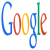```                //不知道是不是楼主的意思
String a = "46328648326846835684657654876576657652364652442384" ;
String b = "47" ;

int b_len = b.length();

String fa = a.substring(0, a.length() - (b_len + 1 )) ;
String ta = a.substring(a.length() - (b_len + 1 ), a.length());

int na = Integer.parseInt(ta) + Integer.parseInt(b);

String result = fa + String.valueOf(na);

System.out.println(result);```

0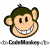```public static void main(String[] args) throws Throwable {
String a = "46328648326846835684657654876576657652364652442384";
String b ="9999999999944876576657652364652442384";
int radix = 10;

BigInteger b1 = new BigInteger(a);
BigInteger b2 = new BigInteger(b);
BigInteger b3 = b1.add(b2);
System.out.println(b3);

int len1 = a.length(),
len2 = b.length();
int len = len1 > len2 ? (len1 + 1) : (len2 + 1);
int[] arr = new int[len];
int[] arr1 = new int[len];
int[] arr2 = new int[len];
int[] real = new int[len];
int index = 0;
while(len1 -- > 0) {
arr1[index] = Character.digit(a.charAt(len1), radix);
index ++;
}
index = 0;
while(len2 -- > 0) {
arr2[index] = Character.digit(b.charAt(len2), radix);
index ++;
}
index = 0;
int dig = 0;
while(index < len) {
int val = arr1[index] + arr2[index] + dig;
if(val > 9) {
dig = 1;
arr[index] = val % radix;
} else {
dig = 0;
arr[index] = val;
}
index ++;
}
index = 0;
while(len -- > 0) {
real[index ++] = arr[len];
}
index = 0;
StringBuffer sb = new StringBuffer(real.length);
while(index < real.length && real[index ++] == 0);
index -= 1;
while(index < real.length) {
sb.append(real[index ++]);
}
System.out.println(sb.toString());
}```

0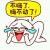```	public static void main(String[] args) {
int[] bj = {1,9,9};
int[] j = {1,1,1};

int[] s = j(bj,j);
for (int i = 0; i < s.length; i++) {
System.out.print(s[i]);
}

}

//bj.length 必须大于等于 j.length
public static int[] j(int[] bj,int[] j){
for (int i = j.length-1,k = bj.length-1; i > -1 ; i--,k--) {

int x = bj[k] + j[i];

if( x > 9){
bj[k] = Integer.parseInt(String.valueOf(x).substring(1, 2));
bj[k-1] = bj[k-1] + 1;
j(bj,new int[j[i]]);
j[i] = 0;
}else{
bj[k] = x;
}
}
return bj;
}```

0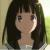0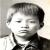#### 引用来自“南湖船老大”的评论